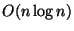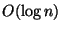Next: Miscellaneous Up: Compression and Representation Previous: MAXIMUM COMMON POINT SET   Index

### MINIMUM RECTANGLE COVER

• INSTANCE: An arbitrary polygon P.

• SOLUTION: A collection of m rectangles whose union is exactly equal to the polygon P.

• MEASURE: Size, i.e. number m of elements, of the collection.

• Good News: Approximable within, where n denotes the number of vertices of the polygon .

• Comment: If the vertices are given as polynomially bounded integer coordinates, then the problem is approximable within. In the case of rectilinear polygons with holes, the rectangular covering is APX-hard . If the solution can contain only squares, then the problem is approximable within 14 .

• Garey and Johnson: SR25

Viggo Kann
2000-03-20